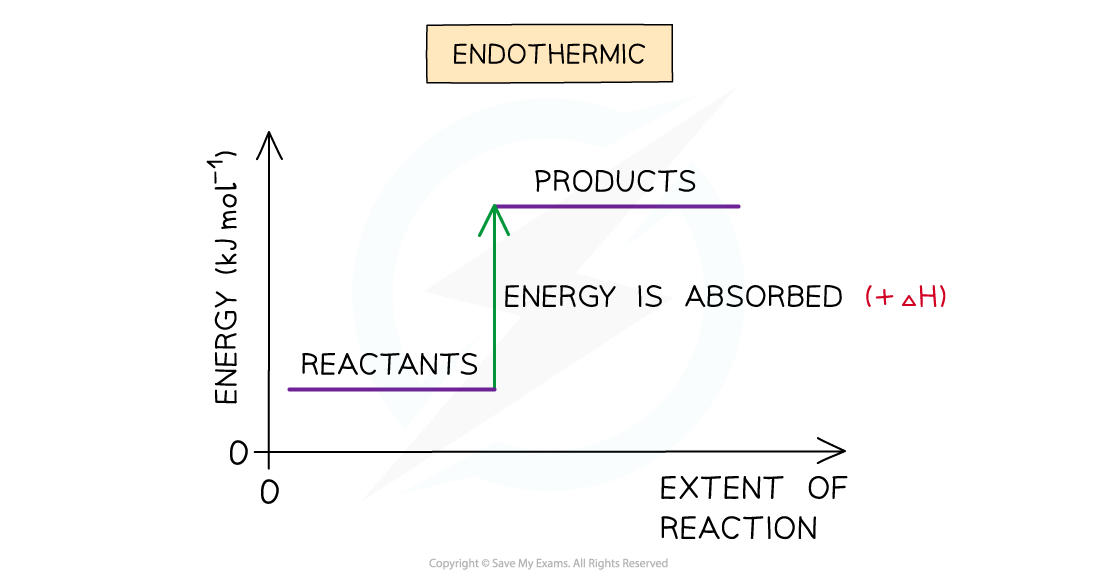# CIE A Level Chemistry复习笔记1.5.1 Enthalpy Change, ΔH

### Enthalpy Changes

• The total chemical energy inside a substance is called the enthalpy (or heat content)
• When chemical reactions take place, changes in chemical energy take place and therefore the enthalpy changes
• An enthalpy change is represented by the symbol ΔH (Δ= change; H = enthalpy)
• An enthalpy change can be positive or negative

#### Exothermic reactions

• A reaction is exothermic when the products have less energy than the reactants
• Heat energy is given off by the reaction to the surroundings
• The temperature of the environment increases - this can be measured on a thermometer
• The temperature of the system decreases
• There is an enthalpy decrease during the reaction so ΔH is negative
• Exothermic reactions are thermodynamically possible (because the enthalpy of the reactants is higher than that of the products)
• However, the rate may be too slow to observe any appreciable reaction
• In this case the reaction is kinetically controlled
• This means the reaction could have a high activation energy which is preventing the reaction from taking place.The enthalpy change during an exothermic reaction

#### Endothermic reactions

• A reaction is endothermic when the products have more energy than the reactants
• Heat energy is absorbed by the reaction from the surroundings
• The temperature of the environment decreases - this can be measured with a thermometer
• The temperature of the system increases
• There is an enthalpy increase during the reaction so ΔH is positiveThe enthalpy change during an endothermic reaction

#### Exam Tip

It is important to specify the physical states of each species in an equation when dealing with enthalpy changes as any changes in state can cause very large changes of enthalpy.For example:Na+Cl- (s) → Na+ (aq) + Cl- (aq)   ΔH = +4 kJ mol-1Na+Cl- (g) → Na+ (g) + Cl- (g)   ΔH = + 500 kJ mol-1Also, remember that the system is the molecules that are reacting (ie. the reaction itself) and the surroundings is everything else (eg. the flask the reaction is taking place in).